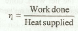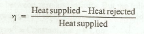### Efficiency of a Cycle

Efficiency of a Cycle may be defined as the ratio of work done to the heat supplied during a cycle. Mathematically, efficiency of a cycle,Since the work done during a cycle is equal to heat supplied minus the heat rejected, the efficiency of a cycle, therefore, may also be expressed asNotes:

1. The efficiency, as given above, is the theoretical efficiency of the cycle. Therefore it is known as theoretical thermal efficiency.

2. It does not take into account the practical losses, which occur in running of the engine.

3. In order to compare the efficiency of the thermodynamic cycles, air is assumed to be the working substance inside the engine cylinder. Moreover, air is assumed to behave as a perfect gas. The efficiency, thus, obtained is known as air standard efficiency. It is also called ideal efficiency.